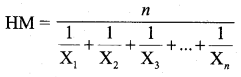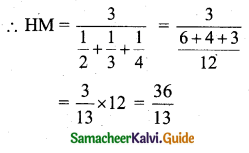Tamilnadu State Board New Syllabus Samcheer Kalvi 11th Business Maths Guide Pdf Chapter 8 Descriptive Statistics and Probability Ex 8.3 Text Book Back Questions and Answers, Notes.

## Tamilnadu Samacheer Kalvi 11th Business Maths Solutions Chapter 8 Descriptive Statistics and Probability Ex 8.3

### Samacheer Kalvi 11th Business Maths Descriptive Statistics and Probability Ex 8.3 Text Book Back Questions and Answers

Question 1.
Which of the following is a positional measure?
(a) Range
(b) Mode
(c) Mean deviation
(d) Percentiles
(d) Percentiles

Question 2.
When calculating the average growth of the economy, the correct mean to use is?
(a) Weighted mean
(b) Arithmetic mean
(c) Geometric mean
(d) Harmonic mean
(c) Geometric mean

Question 3.
When an observation in the data is zero, then its geometric mean is:
(a) Negative
(b) Positive
(c) Zero
(d) Cannot be calculated
(c) Zero

Question 4.
The best measure of central tendency is:
(a) Arithmetic mean
(b) Harmonic mean
(c) Geometric mean
(d) Median
(a) Arithmetic mean

Question 5.
The harmonic mean of the numbers 2, 3, 4 is:
(a) $$\frac{12}{13}$$
(b) 12
(c) $$\frac{36}{13}$$
(d) $$\frac{13}{36}$$
(c) $$\frac{36}{13}$$
Hint:Here n = 3Question 6.
The geometric mean of two numbers 8 and 18 shall be:
(a) 12
(b) 13
(c) 15
(d) 11.08
(a) 12
Hint:Question 7.
The correct relationship among A.M., G.M.and H.M.is:
(a) A.M. < G.M. < H.M.
(b) G.M. > A.M. > H.M.
(c) H.M. > G.M. > A.M.
(d) A.M. > G.M. > H.M.
(d) A.M. > G.M. > H.M.

Question 8.
Harmonic mean is the reciprocal of:
(a) Median of the values.
(b) Geometric mean of the values.
(c) Arithmetic mean of the reciprocal of the values.
(d) Quartiles of the values.
(c) Arithmetic mean of the reciprocal of the values.

Question 9.
Median is same as:
(a) Q1
(b) Q2
(c) Q3
(d) D2
(b) Q2

Question 10.
The median of 10, 14, 11, 9, 8, 12, 6 is:
(a) 10
(b) 12
(c) 14
(d) 9
(a) 10
Hint:
The ascending order of 10, 14, 11, 9, 8, 12, 6 is 6, 8, 9, 10, 11, 12, 14.
In this order middle number is 10.
Median = $$\left(\frac{n+1}{2}\right)^{t h}$$ value
= $$\left(\frac{7+1}{2}\right)^{th}$$
= 10
∴ Median 10.

Question 11.
The mean of the values 11, 12, 13, 14 and 15 is:
(a) 15
(b) 11
(c) 12.5
(d) 13
(d) 13
Hint: The values are in ascending, order.
∴ The mean is the middle value.

Question 12.
If the mean of 1, 2, 3, ….., n is $$\frac{6 n}{11}$$, then the value of n is:
(a) 10
(b) 12
(c) 11
(d) 13
(c) 11
Hint:
The mean of 1, 2, 3,…., n is $$\frac{6 n}{11}$$
i.e., $$\frac{1+2+3+4 \ldots+n}{n}=\frac{6 n}{11}$$
$$\frac{\frac{n(n+1)}{2}}{n}=\frac{6 n}{11}$$
$$\frac{n+1}{2}=\frac{6 n}{11}$$
11(n + 1) = 2 × 6
11n + 11 = 12n
∴ n = 11

Question 13.
The harmonic mean is better than other means if the data are for:
(a) Speed or rates.
(b) Heights or lengths.
(c) Binary values like 0 and 1.
(d) Ratios or proportions.
(a) Speed or rates

Question 14.
The first quartile is also known as:
(a) median
(b) lower quartile
(c) mode
(d) third decile
(b) lower quartile

Question 15.
If Q1 = 30 and Q3 = 50, the coefficient of quartile deviation is:
(a) 20
(b) 40
(c) 10
(d) 0.25
(d) 0.25
Hint:
Coefficient of quartile deviation is = $$\frac{Q_{3}-Q_{1}}{Q_{3}+Q_{1}}$$
= $$\frac{50-30}{50+30}$$
= $$\frac{20}{40}$$
= 0.25

Question 16.
If median = 45 and its coefficient is 0.25, then the mean deviation about median is:
(a) 11.25
(b) 180
(c) 0.0056
(d) 45
(a) 11.25
Hint:
Coefficient of M.D = $$\frac{\mathrm{MD}}{\mathrm{Median}}$$
MD = Coefficient of MD × Median
= 0.25 × 45
= 11.25

Question 17.
The two events A and B are mutually exclusive if:
(a) P(A ∩ B) = 0
(b) P(A ∩ B) = 1
(c) P(A ∪ B) = 0
(d) P(A ∪ B) = 1
(a) P(A ∩ B) = 0

Question 18.
The events A and B are independent if:
(a) P(A ∩ B) = 0
(b) P(A ∩ B) = P(A) × P(B)
(c) P(A ∩ B) = P(A) + P(B)
(d) P(A ∪ B) = P(A) × P(B)
(b) P(A ∩ B) = P(A) × P(B)

Question 19.
If two events A and B are dependent then the conditional probability of P(B/A) is:
(a) P(A) P(B/A)
(b) $$\frac{P(A \cap B)}{P(B)}$$
(c) $$\frac{P(A \cap B)}{P(A)}$$
(d) P(A) P(A/B)
(c) $$\frac{P(A \cap B)}{P(A)}$$

Question 20.
The probability of drawing a spade from a pack of card is:
(a) $$\frac{1}{52}$$
(b) $$\frac{1}{13}$$
(c) $$\frac{4}{13}$$
(d) $$\frac{1}{4}$$
(d) $$\frac{1}{4}$$
Hint:
Number of spade cards is 13.
Total number of cards in pack = 52
Probability of drawing a spade card is = $$\frac{13}{52}=\frac{1}{4}$$

Question 21.
If the outcome of one event does’not influence another event then the two events are:
(a) Mutually exclusive
(b) Dependent
(c) Not disjoint
(d) Independent
(d) Independent

Question 22.
Let a sample space of an experiment be S = {E1, E2, …., En} then $$\sum_{i=1}^{n} \mathrm{P}\left(\mathrm{E}_{i}\right)$$ is equal to:
(a) 0
(b) 1
(c) $$\frac{1}{2}$$
(d) $$\frac{1}{3}$$
(b) 1
Hint:
Sum of probabilities is 1
i.e., $$\sum_{i=1}^{n} \mathrm{P}\left(\mathrm{E}_{i}\right)=1$$

Question 23.
The probability of obtaining an even prime number on each die, when a pair of dice is rolled is:
(a) $$\frac{1}{36}$$
(b) 0
(c) $$\frac{1}{3}$$
(d) $$\frac{1}{6}$$
(a) $$\frac{1}{36}$$
Hint:
When a pair of dice is rolled number of elements in the sample space is 36.
2 is the only even prime number. (2, 2) is the only event of even prime number on both dice.
Required probabilities $$\frac{1}{36}$$.

Question 24.
Probability of an impossible event is:
(a) 1
(b) 0
(c) 0.2
(d) 0.5